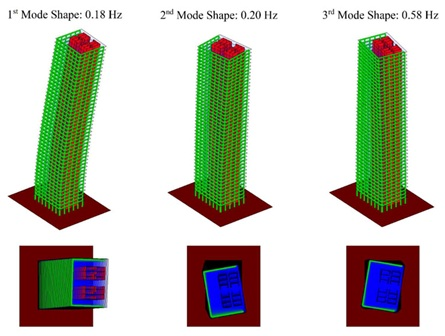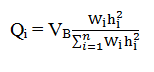Search

# Seismic Method of Analysis

Posted in Earthquake Engineering |Email This Post |

INTRODUCTION
Seismic method of analysis is done in order to ascertain the various responses of buildings during earthquake and also to adopt the retrofitting of structures. It is an important tool for earthquake prone areas like Japan, North-East of India, Nepal, Philippines, and many more. This method of analysis is also important for design of elements of RCC buildings like beam, column, slab which are designed in accordance to IS 13920:2016. The seismic forces are dynamic in nature and these forces are tested for load carrying capacity, ductility, dampness, stiffness and mass. IS 1893:2016 is used to carry out seismic analysis of multi-storeyed building.

TYPES OF SESIMIC ANALYSIS

This method is divided into five categories. These are-
1. Equivalent Static Analysis – In this analysis, an array of forces is used to represent the consequence of earthquake ground motion. It follows the assumption that the building is responsive in its fundamental mode. This is applicable for low rise building and the building which do not rotate significantly about its axis. Further researches have been made to increase its application to high rise buildings and low level of rotation about its axis.

2. Response Spectrum of Analysis – This analysis is carried out when modes other than the fundamental one significantly affect the response of the structure. Here, the response of Multiple-Degree-of-Freedom System (MDOF) is represented as the superposition of modal response where each modal response is determined from the spectral analysis of Single-Degree-of-Freedom-System (SDOF). All these are then amalgamated to calculate the total response.

3. Linear Dynamic Analysis – In circumstances where structures are either too irregular or too tall, the response spectrum analysis is no longer applicable and more complex analysis is often required, such as non-linear static analysis or dynamic analysis. This analysis can be performed either by mode superposition method or response spectrum method and elastic history time method. This analysis generates higher vibration modes and actual distribution of forces in the elastic range in a good way. The difference between Linear Static Analysis and Dynamic Static analysis is the force distribution along the height of the structure and the level of force. Apart from this, the response of the structure to ground motion is calculated in the domain of time, and hence all phase information is maintained.

4. Non-Linear Static Analysis – It is an improvement of linear static or dynamic analysis as it allows the inelastic behaviour of the structure. But one thing is unchanged as it assumes a set of static incremental lateral load over the height of the structure. This analysis is also known as “pushover” analysis. A pattern of forces is applied to a structural model including non-linear properties and the total force is plotted with reference to displacement to depict a capacity curve. This can then be combined with a demand curve (typically in the form of an Acceleration-Displacement Response Spectrum (ADRS)) hence reducing the problem to an SDOF System. Moreover, this analysis is simpler, gives information on strength, deformation, ductility and the distribution of demands. Along with advantages it has some limitation like it neglects the variation in loading patterns and also the effect of resonance and higher modes influence on buildings.

5. Non-Linear Dynamic Analysis – This analysis is based on the direct numerical integration of the motion differential equations by taking into consideration the elasto plastic deformation of the structure element. Its advantage involves producing results with low uncertainty as well as analysis based on time domain. Also, this method considers the amplification due to resonance, changes in displacements at various levels of buildings and increase of motion duration.Fig 1 : Structural Analysis of a High rise building
COURTESY: BENTHAM OPEN

PROCEDURE INVOLVED IN THESE TYPES
Under these analysis discussed above, various procedures are involved so as to design buildings as per seismic conditions to protect those buildings from getting damaged during earthquake. Some of the procedures are –

• Equivalent Static Analysis
1. Firstly, the calculation of lumped masses into various floor levels is done. This involves calculation of masses on roofs and other floors taking into consideration the weight imposed by walls, columns, beams, floors, infills and slabs.

2. Then fundamental natural period is determined using the formula

Ta = 0.075 x h0.75

Where, Ta is the fundamental natural period of vibration in seconds and h is the height of building in meters. This is as per Cl 7.6.2 of IS 1893 Part 1: 2016.

3. As per Cl 7.6.1. of IS 1893 Part 1: 2016, we calculate Base Shear, VB = Ah

4. Then the design Base Shear is distributed along the height of the building as per expression:Qi = Design lateral forces at level i,
Wi = Seismic weights of the floor i
hi = Height of the floor i
n = Number of stories

• Response Spectrum of Analysis
1. First Eigenvalues and Eigenvectors are determined.
2. Then modal participation factors are determined.
3. Modal masses are determined.
4. Lateral force at each floor in each mode is determined.
5. Storey shear forces in each mode is determined.
6. Storey shear force due to all modes are determined.
7. At last Lateral forces at each storey is determined by square root of sum of squares (SRSS) and complete quadratic combination (CQC).

• Linear Dynamic Analysis
1. Firstly, calculation of modal matrix is done.
2. Effective force vector is then calculated.
3. Displacement response in normal coordinate is calculated.
4. Displacement response in physical coordinates is determined.
5. Effective earthquake response forces are determined at each storey.
6. Calculation of Storey Shear is done.
7. At last, maximum response is calculated.

• Non-Linear Static Analysis
1. Firstly, magnitude of lateral load is increased in monolithical manner establishing a predestined pattern of distribution.
2. Building is displaced till it collapses.
3. The relation between base shear and control node displacement is plotted by observation of sequence of cracking, plastic hinging and failure of structural components.
4. Seismic demands are calculated at target displacement level.
5. At last, the seismic demand is then compared to corresponding structural capacity to know the performance of structure.

• Non- Linear Dynamic Analysis
1. Representing the design earthquake, an earthquake record is selected.
2. It is digitized as series of small intervals of time of around 1/40 to 1/25 of a second.
3. Lumped mass at each floor is used to set up mathematical modelling.
4. Digitized record is applied to model as accelerations at the base of structure.
5. The equations of motions are then investigated with software that depicts complete record of acceleration, velocity and displacement of each lumped mass level at each interval.

CONCLUSION
Various approaches are there to ascertain the effect of earthquakes on buildings but these analysis have limitations too. Researches have been made to modify these analysis to extend its application to high rise as well as low buildings simultaneously. For now particular analysis are carried out as per suitability.

REFERENCES
• Wikipedia, “Seismic Analysis” – https://en.wikipedia.org/wiki/Seismic_analysis
• Slideshare, “Seismic Analysis” – https://www.slideshare.net/Krishnagnr/seismic-analysis-68749433
• Pankaj Agarwal, Manish Shrikhande, “Earthquake Resistant Design of Structures”, PHI Learning Pvt. Ltd.

More Entries :